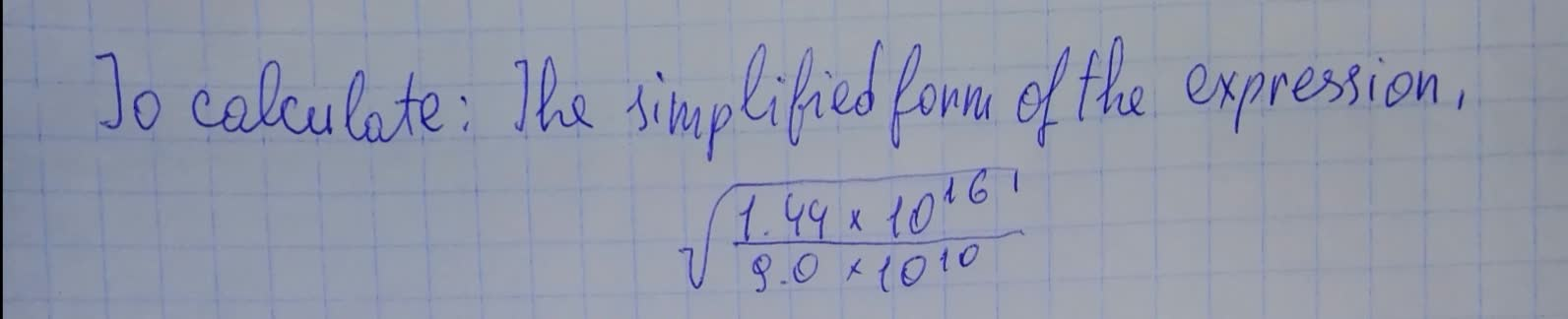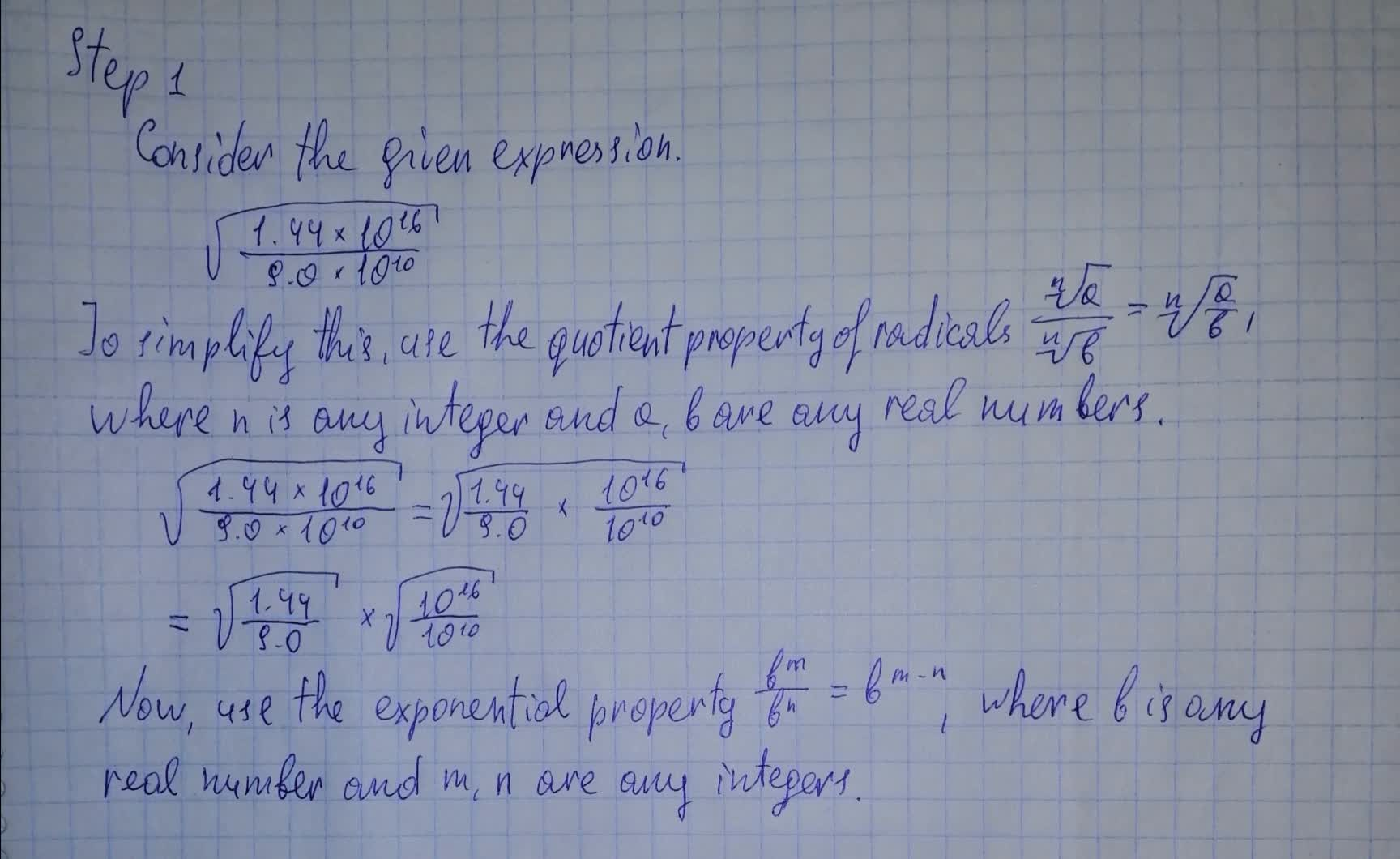To calculate: The simplified form of the expression , \sqrt{\frac{1.44 \times 10^{16}}{9.0 \times 10^{10}}}pancha3 2021-08-14 Answered

To calculate: The simplified form of the expression , $$\displaystyle\sqrt{{{\frac{{{1.44}\times{10}^{{{16}}}}}{{{9.0}\times{10}^{{{10}}}}}}}}$$• Questions are typically answered in as fast as 30 minutes

Solve your problem for the price of one coffee

• Math expert for every subject
• Pay only if we can solve itBentley Leach

Explanation how to solve: